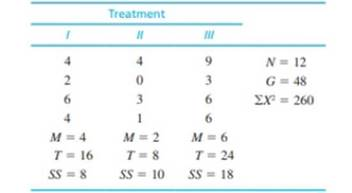Chapter 12, Problem 9PEssentials of Statistics for the B...

8th Edition
Frederick J Gravetter + 1 other
ISBN: 9781133956570

Solutions

Chapter
SectionEssentials of Statistics for the B...

8th Edition
Frederick J Gravetter + 1 other
ISBN: 9781133956570
Textbook Problem

For the preceding problem you should find that there are significant differences among the three treatments. One reason for the significance is that the sample variances are relatively small. To create the following data, we kept the same sample means that appeared in problem 8 but increased the SS values within each sample.a. Calculate the sample variance for each of the three samples. Describe how these sample variances compare with those from problem 8.b. Predict how the increase in sample variance should influence the outcome of the analysis. That is, how will the F-ratio for these data compare with the value obtained in problem 8?c. Use an ANOVA with α = .05 to determine whether there are any significant differences among the three treatment means. (Does your answer agree with your prediction in part b?)

a.

To determine

To find: The sample variances.

To compare: The sample variances with sample variances of problem 8.

Explanation

Given info:

The data shows the values of independent-measures of the three treatment conditions.

Calculation:

Let k represents the numbers of treatments. Then,

k=3

For treatment 1 n=4,SS=8

Let S12 is the sample variance for treatment 1. Then,

S12=SSn1=841=83=2.67

Thus, the sample variance for treatment 1 is 2.67.

For treatment 2:

n=4,SS=10

Let S22 is the sample variance for treatment 2

b.

To determine

To explain: The outcome of the analysis.

c.

To determine

To test: Whether there are significant difference between there treatments means.

Still sussing out bartleby?

Check out a sample textbook solution.

See a sample solution

The Solution to Your Study Problems

Bartleby provides explanations to thousands of textbook problems written by our experts, many with advanced degrees!

Get Started

In Exercises 75-98, perform the indicated operations and/or simplify each expression. 78. 3(2a b) 4(b 2a)

Applied Calculus for the Managerial, Life, and Social Sciences: A Brief Approach

Find if , y = sin 2t.

Study Guide for Stewart's Single Variable Calculus: Early Transcendentals, 8th

True or False: i + j is a unit vector in the direction of 5i + 5j.

Study Guide for Stewart's Multivariable Calculus, 8th